8. THEORY OF SPECTRA OF THE ATOMS AND THE IONS

The development of the theory of spectroscopy was initiated by Balmer-Rydberg and Niels Bohr when they were able to calculate the spectrum of the hydrogen atom , . Schoedinger’s equation was a climax of the theory of spectroscopy , . It gave the possibility to calculate the spectra of all hydrogen-like atoms (the atoms with one electron). But the possibilities of Schroeginger’s equation were very limited. The spectra of all further electrons (beginning from the atomic nucleus) are calculated with the help of Schroedinger’s equation no more. As it has been noted in the fundamental work , in these cases the approximate methods are implemented in order to calculate the spectra of the atoms and the ions.

The main drawback of Schroedinger’s equation is in the fact that it does not give the opportunity to determine the exact position of the electron in the atom, it shows only the density of its presence in this or that field. Empirical character of the approximate methods excludes the possibility of the exact determination of the position of the electron in the atom and makes the formation of the notions concerning its interaction with the atomic nucleus difficult. Due to it, in modern quantum physics the electrons are distributed along the shells, levels and sublevels.

One should note a large work carried out by the spectroscopists - experimenters , , , , . They have measured the characteristics of several hundred thousands spectral lines, but there is no theory, which could allow to calculate these spectra. But the models of the photon and the electron, the main participants of the process of formation of the spectra of the atoms and ions found by us, give the possibility to begin the elaboration of such theory.

As both the photon and the electron have spins equal to Planck’s constant, which is vector quantity, the rules of addition and subtraction of energies of the photons and the electrons should be based on the rules of  vector algebra .

8.1. Spin of the Photon and the Electron

In quantum physics, the notion of spin in  characterizes the rotation of the particles. According to Maxwell theory and the electromagnetic wave model originating from it, the spin of the photon is considered to be equal to a unit and is directed along the trajectory of its motion. The values ±1/2 are ascribed to spin of the electron, and the direction is perpendicular to its orbital plane in the atom . Actually, it is an orbital spin of the electron.

In classical mechanics, the concept of moment of momentum (angular momentum) of the body in relation to an axis, which cross its center of mass, corresponds to the concept of spin. We have already shown that energy of the photon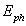and energy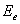of the free electron is determined according to the identical formulas: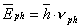(198)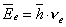(199)

As during absorption or emission of the photons by the electrons their energies are added or subtracted and as they are vector values, this addition and subtraction should be carried out according to the rules of  vector algebra.

From the physical point of view when the photon is absorbed by the electron, their axis of rotation should be parallel. It should originate from the mathematical models of the formation of the spectra as well.

Let us analyse only one energy transition of the electron in the hydrogen atom. Binding energy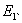of the electron while its staying at the first energy level of this atom is equal to ionization energy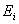of the hydrogen atom, i.e.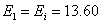electron volt (eV). When the electron absorbs the photon with energy of 10.20 eV and goes to the second energy level, energy of its connection with the nucleus is reduced and becomes equal to 3.40 eV. Naturally, when the photon is absorbed by the electron, their energies are added, and we should write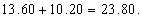(200)

But this result conflicts with the experiment, which points out to the fact that binding energy of the electron with the nucleus after the photon’s absorption is not increased, it s decreased, and it becomes equal to 3.40 eV, not 23,80 eV. That’s why the previous ratio should be written in the following way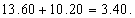(201)

In order to liquidate a contradiction in the formula (201), it has been agreed that energy of the electron in the atom should be considered as negative one, and the formula (201) should be written in the following way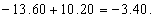(202)

But it is very difficult to agree. The thing is that the electron in the atom has potential component and kinetic component of its full energy. The above-mentioned agreement is acceptable for potential energy, but it cannot be spread to kinetic energy. That’s why it is necessary to try to find more convincing proof of reasonableness of the agreement being reflected in the formula (202).

First of all, there is no complete energyof the electron in the formula (202). The value 13.60 eV is equal to ionization energy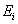of the hydrogen atom. The sense of this energy is in the fact that if the electron absorbs the photon with energy of 13.60 eV, it will lose the connection with the nucleus after it and will become free. It means that the value of 13.60 eV corresponds to binding energy of the electron with the nucleus of the hydrogen atom at the moment when it is on the first energy level. Energy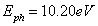is energy of the absorbed photon, which provides a transition of the electron to the second energy level, that’s why energy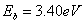, which is equal to difference 13.60-10.20 = 3.40 eV, corresponds to binding energy of the electron with the nucleus of the atom at the moment of its stay at the second energy level .

Let us introduce full energyof the free electron into the equation (202) .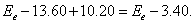(203)

We should remind that 13.60 eV is energy of ionization of the hydrogen atom. It corresponds to binding energy of the electron with the proton at the time when the electron is on the first energy level, and 3.40 eV is binding energy of the electron with the proton, which corresponds to the second energy of the electron; 10.20 eV is energy of the absorbed photon. We can remove valuefrom the equation (203); due to it the equation is not changed, and it will assume the similitude of the formula (202). It is clear that energy of the electron in the atom is a positive value, and the equation (202) reflects the change of  binding energies of the electron only during its energy transitions, and minuses before the values 13.60 and 3.40 mean not negativity of energy, but the process of subtraction of energy being spent for the connection of the electron with the proton .

It is clear that at the time of the stay of the electron on the first energy level in the hydrogen atom its full energyis reduced by energy value 13.60 eV of its binding with the nucleus. When the photon with energyis absorbed, binding energy of the electron with the nucleus is reduced up to 13.60-10.20=3.40 eV. As it is clear, the law of conservation of energy is strictly observed in relation (203). Let us write similar relations for transition of the electron from the first to the third energy level and to the fourth one.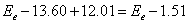,                                            (204)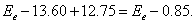(205)

It is easy to note that as the electron is pulled out from the atomic nucleus, its binding energy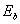with the nucleus is changed according to dependence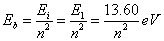,                                                (206)

where n=1, 2, 3 ….. is a number of the energy level of the electron in the atom, the main quantum number.

This is a mathematical model of the law of the change of binding energy with the nucleus of the hydrogen atom and the hydrogen-type atoms. Let us pay attention to the fact that in this case ionization energyis equal to binding energy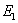of the electron with the nucleus corresponding to the first energy level.

The law of formation of the absorption spectra of the atom of hydrogen and hydrogen-type atoms originates from the relations (202), (203), (204), (205) and (206).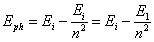(207)

As the spectral lines of absorption coincide with the spectral lines of emission, the mathematical model of the law of radiation should be the same as the one of the law of absorption. It is natural that it does not radiate at the time when the electron is on the first energy level, because this level is the ultimate one for it. But if it is on the second energy level, it can emit the photon with energy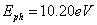. In this case the equation of the radiation process will be written in the following way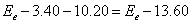.                                                  (208)

When the electron is on the third (n=3) energy level and the fourth (n=4) one, it has binding energies with the nucleus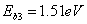and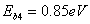. When the electron goes from the third energy level and the fourth one to the first level, it will emit the photons with energies: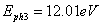and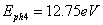, and the equations of these processes will be written in the same way: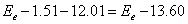,                                                  (209)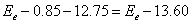.                                                 (210)

# In general, these ratios will be written in the following way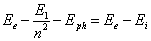.                                                         (211)

If we reduce byand convert, we’ll find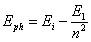,                                                             (212)

It coincides completely with the equation (207). Thus, the same mathematical model of the laws of radiation and absorption of the photons by the electrons during their energy transitions in the atoms originates from the equations of absorption (203), (204) and (205) and radiation (208), (209) and (210).

Now let us consider the physical sense of energies being included in the law (207) of formation of the spectra of the atoms and the ions.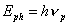is energy of the absorbed or emitted photon.is ionization energy, which is equal to energy of the photon after it being absorbed the electron loses the connection with the nucleus and becomes free. It means that ionization energy is determined according to the same relations as energy of the photon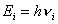. Binding energies of the electron with the atomic nucleus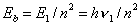(213)

are equal to the energies of the photons. For example, in the hydrogen atom the binding energy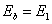of the electron with the atomic nucleus, which corresponds to the first energy level, is equal to energy of its ionization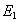. That’s why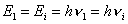. If it is taken into consideration, the mathematical model of the law of emission and absorption (207) of the photons by the electrons during their energy transitions in the atoms can be written in the following way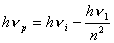.                                                                (214)

Or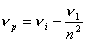(215)

We have got the mathematical model of the law of formation of the spectra of the atoms and the ions, which includes only frequencies of the photons being absorbed or emitted, i.e. frequencies of rotation of the photons in relation to their axes. And where is frequency of rotation of the electron round the nucleus of the atom? None. The energy model of this law (207) has no energy, which corresponds to the orbital motion of the electron. This is an amazing fact. For nearly one hundred years we have supposed that the electron in the atom rotates round the nucleus like a planet round the Sun. The law of formation of the spectra of the atoms and ions (207), (212), (215), which describes energy transitions of the electron in the hydrogen atom, denies the orbital motion of the electron. This law contains no energy, which corresponds to the orbital motion of the electron; this means that it has no such motion. This amazing conclusion makes us think about many things, first of all, about haste in reception of the results of interpretation of many experiments.

The Foundations of Physchemistry of Microworld

Ó2003 Kanarev Ph. M.

Internet Version - http://book.physchemistry.innoplaza.net

<< Back to Physchemistry Book Index